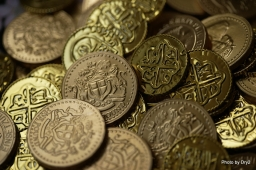# The inheritance

Dad initially decided to bequeath the money to his two sons so that they would share it 8:7 (older and younger). Then he changed his mind and divided the same amount in the ratio of 11:9 (older and younger). This step increased the inheritance value for the older son by 925 tolars.

How much did the father send to both sons together?

x =  49500

### Step-by-step explanation:Did you find an error or inaccuracy? Feel free to write us. Thank you!

Tips for related online calculators
Need help calculating sum, simplifying, or multiplying fractions? Try our fraction calculator.
Check out our ratio calculator.
Do you have a linear equation or system of equations and looking for its solution? Or do you have a quadratic equation?